Mathematical Modeling of Batch Antibiotic Production: Comparative Analysis between Phenomenological and Empirical Approaches | OMICS International
Journal of Bioprocessing & Biotechniques

Like us on:

All submissions of the EM system will be redirected to Online Manuscript Submission System. Authors are requested to submit articles directly to Online Manuscript Submission System of respective journal.

# Mathematical Modeling of Batch Antibiotic Production: Comparative Analysis between Phenomenological and Empirical Approaches

Samuel Conceição Oliveira*

São Paulo State University (Unesp), School of Pharmaceutical Sciences, Araraquara-SP, Brazil

*Corresponding Author:
Samuel Conceição Oliveira
São Paulo State University (Unesp), School of Pharmaceutical Sciences
Rodovia Araraquara-Jaú km 01, Araraquara-SP, Brazil
Tel: +55 16 33014646
E-mail: [email protected]

Received Date: December 09, 2016; Accepted Date: January 30, 2017; Published Date: January 31, 2017

Citation: Oliveira SC (2017) Mathematical Modeling of Batch Antibiotic Production: Comparative Analysis between Phenomenological and Empirical Approaches. J Bioprocess Biotech 7:298. doi: 10.4172/2155-9821.1000298

Copyright: © 2017 Oliveira SC. This is an open-access article distributed under the terms of the Creative Commons Attribution License, which permits unrestricted use, distribution, and reproduction in any medium, provided the original author and source are credited.

Visit for more related articles at Journal of Bioprocessing & Biotechniques

#### Abstract

The batch production of antibiotic by Penicillium chrysogenum is modeled using phenomenological and empirical approaches. In the phenomenological approach, the fungal growth is described by the logistic law, a substrateindependent model for microorganism population dynamics. In addition, the production of penicillin is also modeled considering that the formation of antibiotic is not associated with cell growth and that the product is degraded by hydrolysis according to first-order kinetics. From two sets of experimental data extracted from the literature regarding the batch cultivation of the fungus under different initial cell concentrations (0.18 and 0.40%-DW), it was possible to estimate the model parameters using one of the sets, while the model validation test was performed using the other set. The phenomenological model satisfactorily described the behavior trend of the modeled state variables; however, there is need to obtain a larger amount of experimental data in more diverse initial conditions to achieve a better estimation of parameters and a better description of the bioprocess phases. In the empirical approach, sigmoidal models were adjusted to describe the temporal profiles of cell and product concentrations. The empirical models were successful in describing the bioprocess behavior and appeared quite viable for the modeling of complex bio-systems, such as those comprising the production of antibiotics by filamentous fungi. The parameters of the mathematical models generated by each approach were statistically significant at a confidence level of 95%. Relationships between the growth kinetic parameters of the phenomenological model and those of the empirical model were established.

#### Keywords

Mathematical modeling; Bioprocess; Batch cultivation; Antibiotics; Empirical models; Phenomenological models

#### Introduction

Currently, the development of bioprocesses has sought to meet the ever-growing demands of the reliability and reproducibility of final products, which has boosted the need to improve the monitoring and control of such processes, a context in which mathematical modeling and simulation have taken on important roles.

Within the extensive list of products obtained through biological processes, antibiotics have become a milestone in the history of humanity, allowing for the treatment of a wide range of infectious and cancerous diseases . Among the most commonly commercialized antibiotics worldwide are β-lactam antibiotics, which represent nearly 60% of the world market in this segment, especially penicillin and cephalosporin, due to their clinical importance .

Natural penicillin and cephalosporin are produced industrially through bioprocesses, employing fungus strains of Penicillium and Cephalosporium genuses. Considering that these fungi are filamentous and strictly aerobic, the bioprocess is generally conducted in aerated stirred tank reactors, which are submitted to high stirring speeds to increase the oxygen transfer from the gas phase to the liquid medium .

Although specific antibiotics have proven to be effective in the treatment of diseases, some of these are still not sold in the market due to the low productivity of the bioprocess and to the difficulty in the purification stage, which leads to a high cost for medications . In these cases, the optimization of the production and bio-product recovery processes become necessary, and mathematical modeling is a modern and powerful tool for this end [5,6].

Mathematical modeling can be defined as the process of conceiving and formulating the set of equations that represent the bioprocess. Clearly, the complete description of all of the pathways and metabolic interactions that occur in a biological process would be a virtually impossible task . According to Sinclair and Kristiansen , upon modeling a fermentation process, one should consider only relevant aspects of interest. In this sense, a model would represent a series of relations among variables of interest of a given system under study.

Depending on the degree of knowledge about the system, mathematical modeling can be performed according to an empirical or a phenomenological approach to the bioprocess.

The phenomenological approach attempts to describe the main phenomena involved in the process, using the basic principles of mass, energy, and momentum conservation; constitutive equations, including kinetic expressions for reaction rates and relationships of thermodynamic equilibrium, among others; in addition to the formulation of simplifying hypotheses and of initial and boundary conditions, when necessary.

Generally, the phenomenological modeling of bioprocesses is performed according to a non-segregated and non-structured approach to the microbial population, that is, the cell population is considered to be homogeneous, with cells presenting the same characteristics of age, size, and behavior, and representing the cell material for a sole variable, the cell mass, or the number of cells, without considering the variations in the concentration of intracellular components for the kinetic modeling of the process .

In its great majority, the bioprocesses of antibiotic production are conducted in homogeneous reactors, and the mathematical models associated with these processes are almost completely formulated under isothermal conditions, including only the mass balances of each component. Such balances are represented by ordinary differential equations, which are solved by numerical integration starting from known initial conditions for the modeled variables.

In the empirical approach, the bioprocess is seen as a black box, completely ignoring the mechanisms of cause and effect between the process’s input and output variables. The output variables are correlated empirically with the input variables by means of transfer functions, which may include polynomial, exponential, logarithmic, hyperbolic, sigmoidal models, among other such adjustment models. By contrast, empirical statistical models are based on the planning of experiments and on the theory of regression, while neural network models are based on artificial intelligence concepts and are commonly used to describe complex dynamic systems, such as bioprocesses.

Clearly, empirical models cannot be compared to phenomenological models when the aim is to acquire detailed knowledge on the main phenomena involved in the bioprocess. However, in the cases in which a phenomenological mathematical description is not strictly necessary, the empirical approach can be employed so long as no impairment is caused to the predictive capacity due to this substitution.

The empirical approach seeks to increase the capacity to analyze complex systems, especially in those situations in which a phenomenological approach becomes impracticable for various reasons. One can cite, for example, the case of many bioprocesses of secondary metabolite production, in which complex biochemical aspects are involved, such as the effects of catabolite repression and the morphological differentiation of the biomass during cultivation, in addition to operational problems related to the oxygen transfer due to the non-Newtonian behavior exhibited by the reactional medium, when filamentous fungi are used [3,4].

In the present study, the batch production of penicillin by Penicillium chrysogenum is modeled according to phenomenological and empirical approaches, aimed at drawing a comparison between the predictive capacities of the mathematical models generated by each approach.

#### Material and Methods

Experimental data

The experimental data used in this study were taken from the literature  and pertain to two batch cultures of Penicillium chrysogenum, performed under carefully controlled conditions for the antibiotic production in reactors of 550 liter capacities, at a temperature of 20ºC, and using different initial cell concentrations. The cultivation time, in both cases, was 190 hours, a typical value for this bioprocess.

Mathematical model

Phenomenological approach: The phenomenological mathematical model used to describe the growth of the penicillinproducing fungus (Penicillium chrysogenum) is based on the logistic law of limited growth [11,12]. In this model, the limitation of the microbial growth is not described explicitly as a function of the variation dynamics of the limiting substrate concentration, but rather implicitly by the introduction of the term (1-X/Xm) in the growth rate equation (rx), which diminishes over time and is nullified when X reaches the maximum value of Xm, a condition in which cell growth ends. The production of penicillin is also modeled when one considers that the antibiotic formation kinetics is given by the Luedeking-Piret model (rp=δrx+βX), with δ=0 and β>0, since that the product is a secondary metabolite, produced mostly after the growth phase [8,13]. Also considered is the commonly observed fact that the product is degraded by hydrolysis to a rate proportional to its own concentration, this process being described by first-order kinetics . The complete model, comprised of two ordinary differential equations corresponding to the mass balances of cell and product in a batch bioreactor, and containing four parameters, is represented by: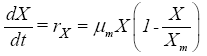(1)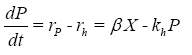(2)

where t is the time (h), X is the cell concentration (%-DW), P is the antibiotic concentration (U mL-1), rX is the cell growth rate (%- DW h-1), rP is the antibiotic production rate (U mL-1 h-1), rh is the product’s hydrolysis rate in (U mL-1 h-1), and μm, Xm, β, and k, Xm, β, and kh are the parameters of the model, according to the following meanings: μm is the maximum specific growth rate (h-1), Xm is the maximum possible cell concentration to be achieved (%-DW), β is the constant of product formation not associated with growth (U mL-1 h-1 (%-DW)-1), and kh is the rate constant for the antibiotic hydrolysis reaction (h-1).

Such parameters were estimated by non-linear regression, using the Marquardt method .

Empirical approach: The empirical mathematical model of the bioprocess consists of independent sigmoidal equations that were adjusted to the experimental data of each variable obtained in the two cultures. The general form of the adjusted sigmoidal equations is the following: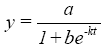(3)

where y represents the cell (X) or the product (P) concentration, t is time, and a, b, k are the equation parameters, which, as they refer to an empirical approach, do not have a phenomenological meaning and were also estimated by non-linear regression, using the Marquardt method .

In Equation 3 the parameter a has a concentration unit, b is dimensionless, and k has an inverse unit of time, i.e. time-1.

#### Results and Discussion

Phenomenological approach

Table 1 shows the estimates of the phenomenological model parameters and their respective standard deviations. One can observe that the standard deviation of μm and Xm are small when compared to the respective values of the parameters, indicating good precision of its estimates, which does not occur with β and kh .

Parameters Estimated values  tc=parameter/standard deviation
μm(h-1) 0.049836 ±0.002138 23.310
Xm(%-DW) 3.6347 ± 0.0906 40.118
β(U mL-1h-1(%-DW )-1 0.020496 ± 0.005501 3.726
kh (h-1) 0.026516 ± 0.009727 2.726

Table 1: Estimated values of the phenomenological model’s kinetic parameters.

The statistical significance of the estimated parameters can be evaluated by comparing the values of t calculated (tc) with the respective tabulated values of t (tt) for (N-p) degrees of freedom and α/2. If tc ≥ tt, the parameter is considered to be significant at a confidence level of (1- α) × 100%. Considering α=0.05 (most commonly adopted significance level), the result is tt (30, 0.025)=2.042. Thus, based on the Student t test, all of the phenomenological model’s parameters are statistically significant at a confidence level of 95%.

Typical values for the parameters were obtained in comparison with values reported in the literature, according to the data presented in Table 2. In this table, the literature-reported values of the parameters are provided at three temperatures , and the units are the same as presented in Table 1. Although the temperature does have an effect on the kinetic parameters, it is possible to have a notion of the values that these can reach.

Parameters Temperature (°C) Estimated values Reported values 
μm 20 0.049836 ± 0.002138 0.04459 ± 0.00479

25 - 0.06267 ± 0.01550

30 - 0.06313 ± 0.01252
Xm 20 3.6347 ± 0.0906 3.861 ± 0.234

25 - 3.273 ± 0.299
30 - 3.139 ± 0.244
β 20 0.020496 ± 0.005501 0.04538 ± 0.02255

25 - 0.09461 ± 0.06964

30 - 0.09281 ± 0.07164
kh 20 0.026516 ± 0.009727 0.06123 ± 0.03396
25 - 0.07546 ± 0.05908
30 - 0.08112 ± 0.06630

Table 2: Estimated and literature-reported values of the phenomenological model’s kinetic parameters for temperatures of 20°C, 25°C and 30°C.

Figure 1 shows the behavior of the model when confronted with the set of experimental data used to estimate the kinetic parameters. According to this figure, one can observe that the mathematical model adequately describes the behavior trend of the modeled state variables (X, P). However, the validation of the model should be performed using a set of experimental data that was not used in the parameter estimation step, so as to perform a rigorous test of the predictive capacity of the model. This procedure was applied, and the results are shown in Figure 2. Analyzing Figure 2, one can observe that the mathematical model describes the behavior trend of the state variables, but fails to predict the cell concentration in the final phase of the bioprocess, most likely because the Xm parameter was estimated by means of data obtained from a culture in which the biomass production was less than that of the culture whose data were used in the model validation. In this sense, the model predictions at the end of the bioprocess for the validation test were systematically lower than the respective experimental data, due to the limiting effect of the Xm parameter. This result points to the need to estimate the model parameters using experimental data obtained under the broadest possible initial conditions in an attempt to reach a precise estimate of the parameter that defines the end of fungal growth. Another possibility is to review the model through the incorporation of new aspects, aimed at improving its predictive capacity.

Figure 1: Behavior of the phenomenological model versus the experimental data used in the parameter estimation step.

Figure 2: Behavior of the phenomenological model versus the experimental data used in the validation step.

Empirical approach

Figures 3 and 4 present the behavior of the sigmoidal models in comparison with the experimental data of each variable for the two cultures. These figures also present the estimated values of the model parameters and their respective standard deviations to provide a notion of the level of precision achieved in the estimates. For all of the adjustments made in this step, according to the Student t test, the estimated parameters proved to be statistically significant at a confidence level of 95%.

Figure 3: Behavior of the empirical sigmoidal models versus the experimental data obtained in the culture, with X0=0.18 %-DW.

Figure 4: Behavior of the empirical sigmoidal models versus the experimental data obtained in the culture, with X0=0.40 %-DW.

Figures 3 and 4 demonstrate very good adjustments of the sigmoidal models to the experimental data of each variable obtained in the two cultures. The empirical models, as they are not based on phenomenological principles, are not reliable enough to make extrapolations, and are thus classified as local models. Hence, the parameters of each model were adjusted locally, aimed at representing the specific conditions of the bioprocess. In this sense, Lau et al.  in a predictive scheduling study of a penicillin bioprocess plant, used Equation 3 to adjust the experimental data of batch antibiotic production and obtained good adjustments.

Relationship between the parameters of the two approaches

Though the parameters of the empirical models present no phenomenological meaning, it is possible, for the cell concentration variable (X), to establish relationships between the parameters of the phenomenological model and the those of the empirical model. In fact, the solution of Equation 1, subject to the initial condition X(0)=X0, is given by [12,17,18]: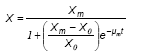(4)

Otherwise, the cell concentration predicted by the empirical model is given by: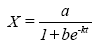(5)

When comparing Equations (4) and (5), one can establish the following relationships between the parameters of the empirical and phenomenological models: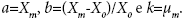An analogous development for the antibiotic concentration variable (P) is not possible, since the solution of Equation (2), after having inserted Equation (4), does not fit the format of Equation (3), thus making it impossible to establish any relationship between the parameters of the two approaches, as had been performed for the cell concentration variable.

Considerations on the advantages of the use of the proposed models

A wide range of studies presented in the literature report on the use of other mathematical resources, such as “intelligent” models based on neural networks, genetic algorithms, and specialist systems to analyze biosystems, such as those studied herein [19-25]. The advantages of the use of the models proposed in the present article lie in the fact that one, of phenomenological nature, is based on the principle of mass conservation and incorporates specific kinetic equations, making it more reliable when making interpolations and extrapolations, as compared to purely empirical models. As it is a non-segregated and non-structured phenomenological model, it becomes robust and can be applied to describe bioprocesses under various operating conditions of temperature, pH, and other such controlled variables [18,26]. In addition, the non-structured models require much less monitoring, which makes them attractive for industrial use . The other model, of empirical nature, is also robust, since, upon readjusting its parameters, it becomes capable of adapting itself to different conditions. This robustness of the models is clear in Figure 5, which presents the adjustments of the phenomenological and empirical models to a new set of data extracted from the literature . According to this figure, one can observe that the proposed models satisfactorily describe the behavior trend of the antibiotic production bioprocess within a different condition. It can also be observed that the empirical model, when compared to the phenomenological model, provide a better description of the cell concentration behavior, while for the antibiotic concentration, the predictions of the two models are quite similar and of good quality. To adjust these models to the new set of data, it was necessary to normalize the antibiotic concentration values, due to the difference in the value scale of X (1 to 49.5 g/L) and P (0 to 1920 U/mL). For this, it was applied the equation P*=P(t)/P(t=10 h), where P(t=10 h) = 193 U/mL. As a consequence of this normalization, the antibiotic’s mass balance equation becomes:

Figure 5: Adjustment of the mathematical models to the new set of data: (a) – phenomenological model (μm=0.12709 h-1, Xm=44.806 g L-1, β*=0.032003 L g-1 h-1, kh =0.028081 h-1) (b) – empirical model (X: a=47.31002 g/L, b=9.08454, c=0.07029 h-1; p*: a=47.1442, b=22.03062, c=0.05577 h-1).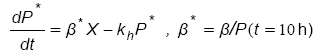(6)

Neural networks are a type of input-output model that allows one to calculate one or more respective system responses (outputs) by means of a defined number of measured input variables . Though advocated as tools that are highly capable of representing complex systems, neural networks have been pushed aside in favor of simpler empirical models, such as those used in the present study, due to the large quantity of experimental data required in its training and validation steps. On the contrary, the empirical models proposed in this study are mathematically simple, contain a reduced number of constants, and thus demand much less experimental and computational efforts to identify its parameters. Moreover, these models can be found in statistical software, such as ORIGIN, and can be easily adjusted from tabulated experimental data. The disadvantage of the models based on genetic algorithms lies in the enormous computational effort needed to process and test the millions of combinations inherent to this type of approach. Models based on specialist systems are known for being nonparametric, conceived through the knowledge of qualified operators (“experts”), which are used to define a set of logical conditional rules that describe the behavior of the process [19,21]. Therefore, these models are qualitative in nature, which is seen as a disadvantage.

#### Conclusion

From the results obtained in this study, one can conclude that the phenomenological model, which incorporates non-structured and nonsegregated kinetics of cell growth and product formation, was able to satisfactorily describe the behavior trend of the state variables during the batch production of antibiotic by the fungus Penicillium chrysogenum. Nevertheless, better estimates of the parameters and/or refinements in the model are warranted in order to expand the model’s validity range.

The bioprocess was also described satisfactorily by an empirical approach in which sigmoidal models were applied to describe the dynamic behavior of cell and product concentrations throughout the bioprocess. The good adjustments obtained in this study highlight the application potential of empirical models to describe complex bioprocesses, as is the case of the production of antibiotics by filamentous fungi in aerated stirred tank reactors.

Comparatively, the empirical models better described the experimental data than did the phenomenological models. However, it should be duly noted that, as these empirical models do not have a phenomenological basis, they serve only to make interpolations within the experimental region in which they were developed. In this sense, extrapolations performed with this type of model should be avoided due to the risk of making imprecise predictions about state variables.

#### Acknowledgement

The author wishes to thank CNPq for their financial support (Protocol number: 455487/2014-6).

#### References

Select your language of interest to view the total content in your interested language

### Article Usage

• Total views: 2332
• [From(publication date):
February-2017 - Aug 24, 2019]
• Breakdown by view type
• HTML page views : 2142
• PDF downloads : 190

## Post your commentCan't read the image? click here to refresh
###### Peer Reviewed Journals

Make the best use of Scientific Research and information from our 700 + peer reviewed, Open Access Journals

International Conferences 2019-20

Meet Inspiring Speakers and Experts at our 3000+ Global Annual Meetings

Top